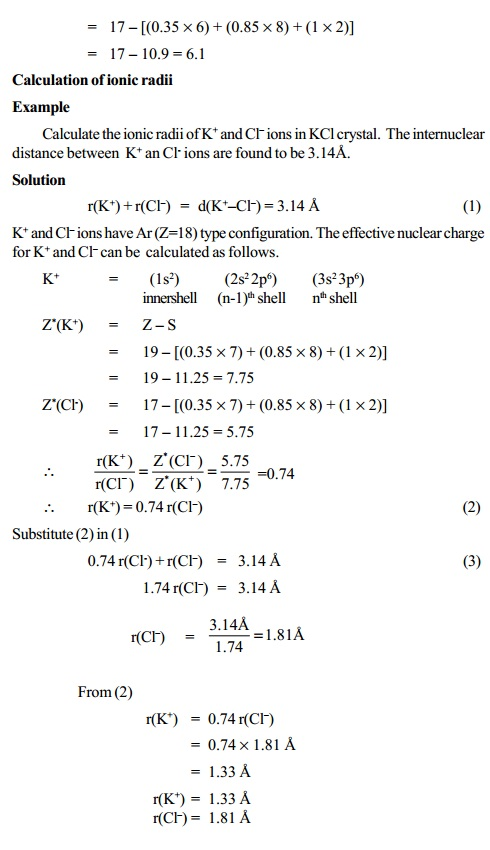Home | | Chemistry | Calculation of ionic radii - Pauling's Method, Slater rules

# Calculation of ionic radii - Pauling's Method, Slater rules

Pauling has calculated the radii of the ions on the basis of the observed internuclear distances in four crystals namely NaF, KCl, RbBr and CsI. In each ionic crystal the cations and anions are isoelectronic with inert gas configuration.

Pauling's Method

Pauling has calculated the radii of the ions on the basis of the observed internuclear distances in four crystals namely NaF, KCl, RbBr and CsI. In each ionic crystal the cations and anions are isoelectronic with inert gas configuration.

NaF crystal : Na+ - 2, 8   F- - 2, 8   Ne  type configuration

KCl crystal : K+ - 2, 8, 8    Cl-   - 2, 8, 8   Ar  type configuration

Further the following two assumptions are made to assign the ionic radii.

i) The cations and anions of an ionic crystal are assumed to be in contact

with each other and hence the sum of their radii will be equal to the inter nuclear distance between them.

r(C+) + r(A-) = d (C+-A-)

where

r(C+) - radius of cation, C+ r(A-)

d(C+-A-) - internuclear distance between C+ and A- ions in C+A- ionic crystal

ii) For a given noble gas configuration, the radius of an ion is inversely proportional to its effective nuclear charge. i.e.

r(C+ ) � = 1/ Z (C+ )

r(A- ) � = 1/ Z (A- )

where,

Z*(C+) & Z*(A-) are the effective nuclear charges of cation (C+) and anion (A-) respectively. On combining

r(C+ ) / r(A- ) = Z*(A- ) / Z*(C+ )

Hence the above two equations (1) & (4) can be used to evaluate the

values of r(C+) and r(A-) provided that the values of d(C+-A-), Z*(C+) and Z*(A-) are known.

Slater rules

The value of screening constant (S) and effective nuclear charge (Z*) can

be calculated by using Slater's rules. According to these rules the value of "S" for a given electron is estimated as follows.

i) Write down the complete electronic configuration of the element and divide the electrons into the following orbital groups starting from the inside of the atom.

(1s) :   (2s, 2p) : (3s, 3p) : (3d) : (4s, 4p) :

(4d) :   (4f)      : (5s, 5p) : (6s, 6p) .......etc.

ii) Select the electron for which the value of S is to be calculated. For this calculation add up the contributions to S for the other electrons according to the following rules.

Contribution to S for each electron of this type

Type of electron

1.           All electrons in groups outside the electron chosen  - 0

2.           All other electrons in the same group as the chosen one (n) - 0.35 (or 0.30 for 1s electron)

3.           All electrons in shell immediately inside (n-1)  - 0.85

All electrons further inside  -  1.00Study Material, Lecturing Notes, Assignment, Reference, Wiki description explanation, brief detail
11th 12th std standard Class Organic Inorganic Physical Chemistry Higher secondary school College Notes : Calculation of ionic radii - Pauling's Method, Slater rules |